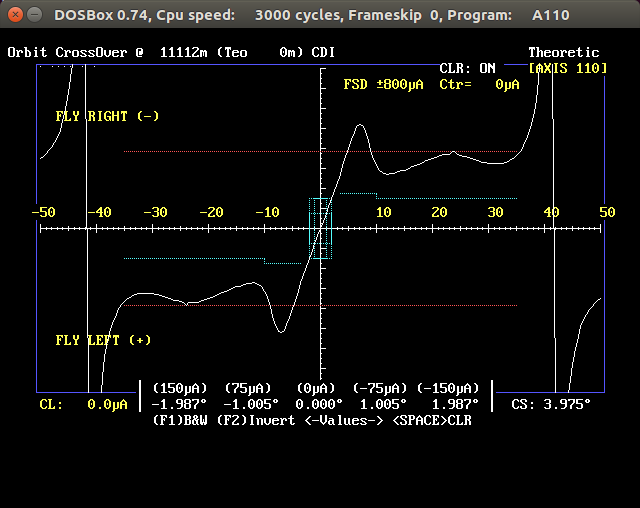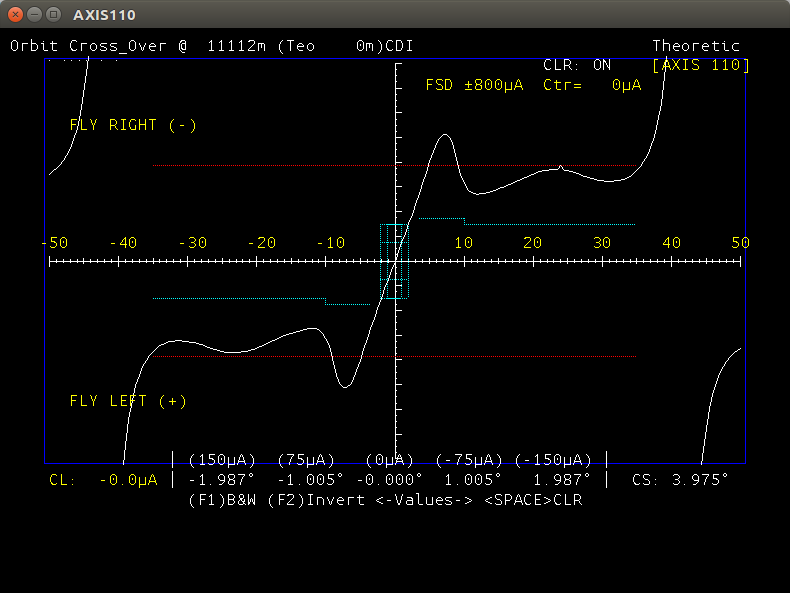# Industrial Case: Timeless automations in an ILS Localiser Simulator from 1980’s

Martin F. Johansen, 2019-01-27

The progsbase tooling and theory has been successfully used to convert about 13,000 lines of code from Turbo Basic to Java. After being converted, further modernizations are within reach and modern development tooling and hardware can be utilized. This industrial case is an example of the theory behind progsbase: That computational code has the potential to last a long time. The computations in this industrial case have already lasted for decades and will now last for many more.

AXIS 110 ILS Localiser Simulator is software with a wide range of computations related to an Airport's Instrument Landing System (ILS). It has been developed by Nordic Air Navigation Consulting (NANCO). The software has been used in more than 25 countries for more than 30 years.

The oldest entry in the development log is from 1979!

The software was written and maintained in Turbo Basic, a compiler and IDE developed by Borland. Here is a screenshot of the Turbo Basic IDE.AXIS 110 had about 13,000 lines of code which is mostly computational. Some of the code is for a textual UI, a graphical UI and some code is for reading and writing files.

Turbo Basic code compiles to a 16-bit x86 DOS executable. Such executables became increasingly difficult to run on Windows, and from Windows XP onwards, AXIS 110 had to be run in DOSBox. Executables made with Turbo Basic are limited to 640 kB RAM, a 80x25 text screen and a 640x480 graphics screen. The file names are limited to 8+3 characters and their encoding to ASCII with DOS extensions.

Needless to say, staying with such old technology is an increasing problem. Still, the software works well and has a good, working and tested implementation of its computations that has stood the test of time.

The question is: How tightly coupled is the program to 16-bit x86 machine code and DOS? The answer is, maybe surprisingly, not very coupled at all.

Here is an excerpt of a computational function involved in computing the scattering of signals:

``````sub CS(C,S,X)
local a,b,d,z

Z=abs(X)
IF Z < 4 then   'Computes C&S from 0   (0 < Z < 4)
C=sqr(Z)
S=Z*C
Z=(4-Z)*(4+Z)
C=C*((((((5.100785E-11*Z+5.244297E-9)*Z+5.451182E-7)*Z_
+3.273308E-5)*Z+1.020418E-3)*Z+1.102544E-2)*Z+1.840965E-1)

S=S*(((((6.677681E-10*Z+5.883158E-8)*Z+5.051141E-6)*Z_
+2.441816E-4)*Z+6.121320E-3)*Z+8.026490E-2)

else  'Computes C&S around 0.5   ( 4 <= Z < infinite)

D=COS(Z)
S=SIN(Z)
Z=4/Z
A=(((((((8.768258E-4*Z-4.169289E-3)*Z+7.970943E-3)*Z-6.792801E-3)_
*Z-3.095341E-4)*Z+5.972151E-3)*Z-1.606428E-5)*Z-2.493322E-2)*Z_
-4.444091E-9
B=((((((-6.633926E-4*Z+3.401409E-3)*Z-7.271690E-3)*Z+7.428246E-3)_
*Z-4.027145E-4)*Z-9.314910E-3)*Z-1.207998E-6)*Z+1.994711E-1

Z=sqr(Z)
C=.5+Z*(D*A+S*B)
S=.5+Z*(S*A-D*B)
end if
end sub
``````

There is little reason this procedure is tightly coupled to 16-bit DOS. It uses floating point arithmetic (addition, multiplication, subtraction and division) and the widely used mathematical operators absolute, square root, sine and cosine.

Here is the converted function:

``````public static double [] cs(double c, double s, double x){
double a = 0d;
double b = 0d;
double d = 0d;
double z = 0d;
z = abs(x);
if(z < 4){
// Computes C&S from 0   (0 < Z < 4)
c = sqrt(z);
s = z * c;
z = (4 - z) * (4 + z);
c = c * ((((((5.100785E-11 * z + 5.244297E-9) * z + 5.451182E-7) * z + 3.273308E-5) * z + 1.020418E-3) * z + 1.102544E-2) * z + 1.840965E-1);
s = s * (((((6.677681E-10 * z + 5.883158E-8) * z + 5.051141E-6) * z + 2.441816E-4) * z + 6.121320E-3) * z + 8.026490E-2);
}else{
// Computes C&S around 0.5   ( 4 <= Z < infinite)
d = cos(z);
s = sin(z);
z = 4 / z;
a = (((((((8.768258E-4 * z - 4.169289E-3) * z + 7.970943E-3) * z - 6.792801E-3) * z - 3.095341E-4) * z + 5.972151E-3) * z - 1.606428E-5) * z - 2.493322E-2) * z - 4.444091E-9;
b = ((((((- 6.633926E-4 * z + 3.401409E-3) * z - 7.271690E-3) * z + 7.428246E-3) * z - 4.027145E-4) * z - 9.314910E-3) * z - 1.207998E-6) * z + 1.994711E-1;
z = sqrt(z);
c = .5 + z * (d * a + s * b);
s = .5 + z * (s * a - d * b);
}

return new double [] {c, s};
}
``````

As can be seen, apart from the updated syntax, there is very little that needed fundamental change: Some functions has a different name in Java, and Java does not have pass-by-reference for primitives, so two variables had to be returned.

## The translation

Over 95% of the code was automatically translated. A new lexer and a new parser was developed for Turbo Basic. The code was read into a slightly extended version of the progsbase model, used by the progsbase tool to automatically generate source code for 12 different programming languages.

Once the entire code base of AXIS 110 had been read in, some static analysis was required to determine the types of the variables and which variables were global. Once this was in place, a Java writer was used, similar to the one found in progsbase, to write out the code base to Java.

The remaining 5% of the code had to be manually edited. Some code used goto, some code used pass-by-reference for primitives and some code used calls no longer available such as interrupts and reading off DOS-specific memory.

## Implementing the Standard Library

Once the translation was done, the code compiled successfully in Java. The original code used the Turbo Basic Library, which at this point was only stubs in Java.

The parts of the library used in AXIS 110 was reading and writing files, drawing a textual UI, taking keyboard input and writing graphics. These were well documented in the Turbo Basic manual and were easy to reimplement in Java.

## The Result

Here is the old textual UI running in DOSBoxHere is the new textual UI running in JavaHere is the old graphics running in DOSBoxHere is the new Graphics running in Java### Improved Graphics Resolution

The AXIS 110 graphics was implemented using vector graphics. This means that when the graphics size is set to the window size of the windowing system of Java, the graphics looks sharper and more detailed. The vector drawing functions draws more pixels: The result looks better and has more details.

### Improved Speed

AXIS 110 now runs in 64-bit Java, and not in the DOSBox emulator. This caused great speed improvements for the computations, more than a thousand times quicker. Computations that before took half-an-hour now takes less than a second.

### Improved Computations

As 64-bit memory now is available, the computations can be done at a higher density. This improved the quality of some of the computations utilizing numeric methods.

### Improved Tooling

Further development can now be done using modern Java development environments, such as IntelliJ IDEA. This enables finding unused functions and code, being able to navigate to a function declaration or computing where a function is used, syntax highlighting, a modern code-editor, static analysis and optimizations, and more.

### Improved Possibilities

Now that the code is in Java, the textual UI can be remade using a modern windowing-system. Also, new libraries only available in modern languages can be utilized.

## Conclusion

Nordic Air Navigation Consulting (NANCO) were impressed with the conversion of the software into Java. This brings new life into the product. Within the code base are quality assured computations that have been used and tested through many years. Converting the computations to a new programming language means keeping the quality while acquiring new possibilities.

There are probably tens of billions of lines of code out there with computations that are still valid, have been thoroughly tested over many years but are written in a now unused programming language and for an unused operating system. The industrial case of converting the AXIS 110 code shows the potential of the progsbase system in preserving tried and tested computational code.

###### Contact Information

We would be more than happy to help you. Our opening hours are 9–15 (CET).

📞 (+47) 93 68 22 77

Nils Bays vei 50, 0876 Oslo, Norway